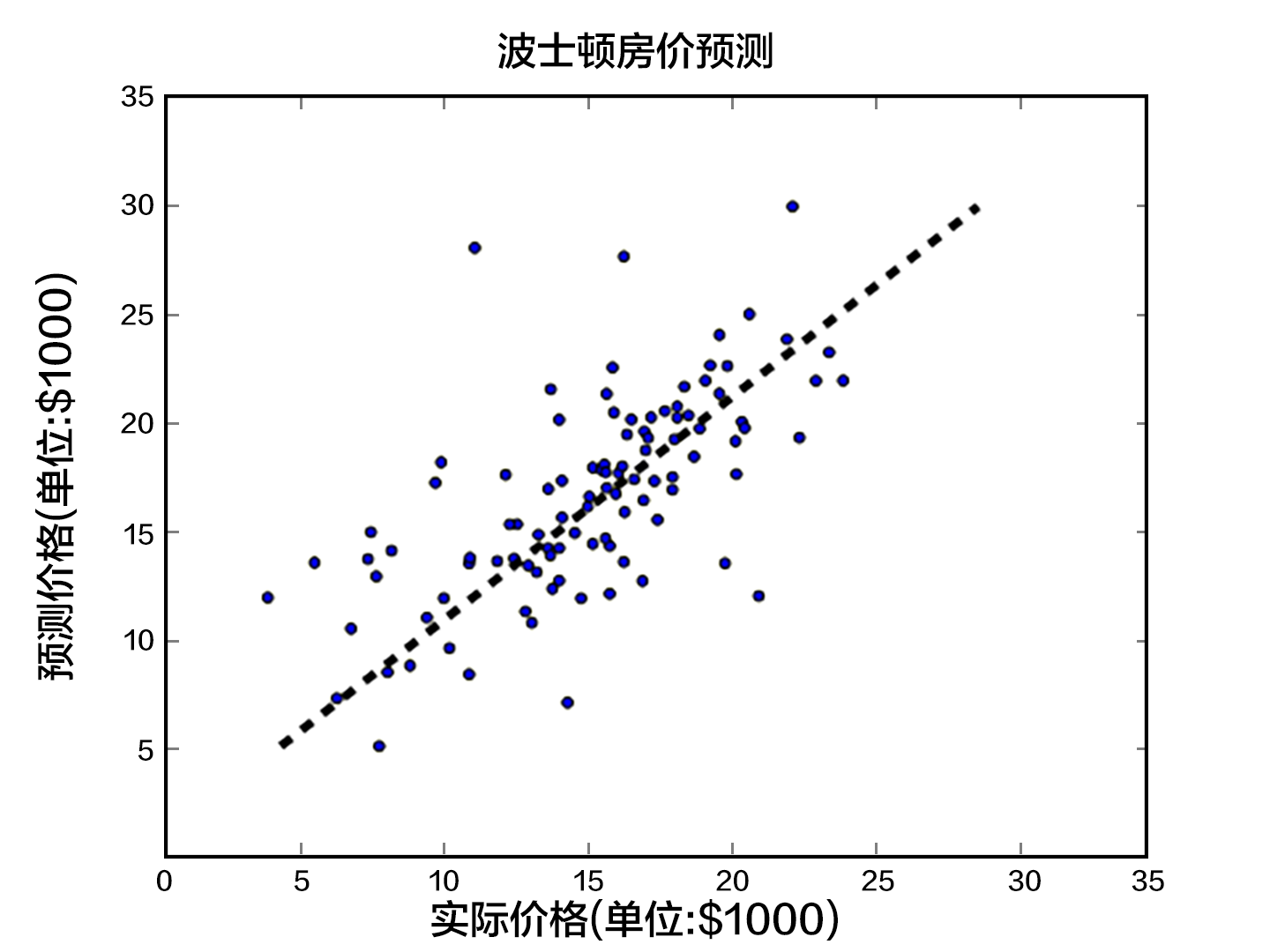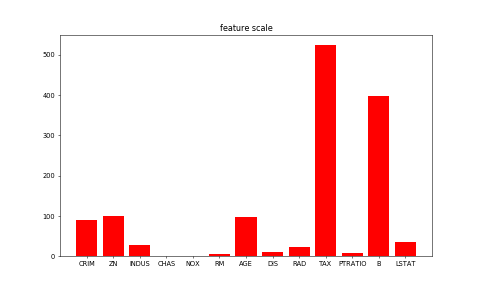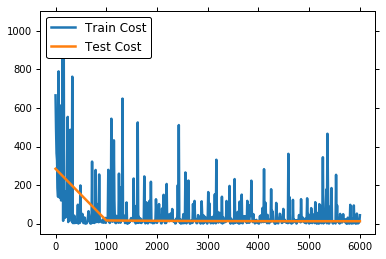- Python 2.7
- Ubuntu 16.04

线性回归

背景介绍

${y}_{i}={\omega }_{1}{x}_{i1}+{\omega }_{2}{x}_{i2}+\dots +{\omega }_{d}{x}_{id}+b,i=1,\dots ,n$

效果展示模型概览

模型定义

$\stackrel{^}{Y}={\omega }_{1}{X}_{1}+{\omega }_{2}{X}_{2}+\dots +{\omega }_{13}{X}_{13}+b$

$\stackrel{^}{Y}$$\hat{Y}$表示模型的预测结果，用来和真实值$Y$$Y$区分。模型要学习的参数即：${\omega }_{1},\dots ,{\omega }_{13},b$$\omega_1, \ldots, \omega_{13}, b$

$MSE=\frac{1}{n}\sum _{i=1}^{n}{\left(\stackrel{^}{{Y}_{i}}-{Y}_{i}\right)}^{2}$

训练过程

1. 初始化参数，其中包括权重${\omega }_{i}$$ω_i$和偏置$b$$b$，对其进行初始化（如 0 均值，1 方差）。
2. 网络正向传播计算网络输出和损失函数。
3. 根据损失函数进行反向误差传播 （backpropagation），将网络误差从输出层依次向前传递, 并更新网络中的参数。
4. 重复 2~3 步骤，直至网络训练误差达到规定的程度或训练轮次达到设定值。

数据集

数据集接口的封装

#coding:utf-8
import os

with_gpu = os.getenv('WITH_GPU', '0') != '0'

#print with_gpu
#print os.getenv('WITH_GPU', '0')
#print os.getenv('WITH_GPU', '1')


2. 数据预处理的过程。

数据集介绍

CRIM 该镇的人均犯罪率 连续值
ZN 占地面积超过25,000平方呎的住宅用地比例 连续值
INDUS 非零售商业用地比例 连续值
CHAS 是否邻近 Charles River 离散值，1=邻近；0=不邻近
NOX 一氧化氮浓度 连续值
RM 每栋房屋的平均客房数 连续值
AGE 1940年之前建成的自用单位比例 连续值
DIS 到波士顿5个就业中心的加权距离 连续值
TAX 全值财产税率 连续值
PTRATIO 学生与教师的比例 连续值
B 1000(BK - 0.63)^2，其中BK为黑人占比 连续值
LSTAT 低收入人群占比 连续值
MEDV 同类房屋价格的中位数 连续值

数据预处理

属性的归一化

- 过大或过小的数值范围会导致计算时的浮点上溢或下溢。
- 不同的数值范围会导致不同属性对模型的重要性不同（至少在训练的初始阶段如此），而这个隐含的假设常常是不合理的。这会对优化的过程造成困难，使训练时间大大的加长。
- 很多的机器学习技巧/模型（例如L1，L2正则项，向量空间模型-Vector Space Model）都基于这样的假设：所有的属性取值都差不多是以0为均值且取值范围相近的。

(deep learning normalization [CN] P141 L1，L2正则项)训练

fit_a_line/trainer.py演示了训练的整体过程。

paddle.init(use_gpu=False, trainer_count=1)

模型配置

# network config
# 定义训练数据 x 根据上述文章描述，前 13 个属性或特征作为 一个样本的训练数据
# 激活函数 使用 Linear() 线性回归 对 y_predict 进行预测
size=1,
# 定义 1 个值作为真实数据的 标签 y
# 使用均方根误差 计算 真实值 y 和预测值 y_predict 之间的损失函数
cost = paddle.layer.square_error_cost(input=y_predict, label=y)

保存网络拓扑

# Save the inference topology to protobuf.
with open("inference_topology.pkl", 'wb') as f:
inference_topology.serialize_for_inference(f)

创建参数

parameters = paddle.parameters.create(cost)

创建Trainer

# create optimizer 优化函数 使用 Momentum 函数
# SGD 随机梯度下降
parameters=parameters,
update_equation=optimizer)

读取数据且打印训练的中间信息

feeding={'x': 0, 'y': 1}

# event_handler to print training and testing info
def event_handler(event):
if event.batch_id % 100 == 0:
print "Pass %d, Batch %d, Cost %f" % (
event.pass_id, event.batch_id, event.cost)

result = trainer.test(
uci_housing.test(), batch_size=2),
feeding=feeding)
print "Test %d, Cost %f" % (event.pass_id, result.cost)
# event_handler to print training and testing info

train_title = "Train cost"
test_title = "Test cost"
cost_ploter = Ploter(train_title, test_title)

step = 0

def event_handler_plot(event):
global step
if step % 10 == 0:  # every 10 batches, record a train cost
cost_ploter.append(train_title, step, event.cost)

if step % 100 == 0: # every 100 batches, record a test cost
result = trainer.test(
uci_housing.test(), batch_size=2),
feeding=feeding)
cost_ploter.append(test_title, step, result.cost)

if step % 100 == 0: # every 100 batches, update cost plot
cost_ploter.plot()

step += 1

if event.pass_id % 10 == 0:
with open('params_pass_%d.tar' % event.pass_id, 'w') as f:
trainer.save_parameter_to_tar(f)

开始训练

trainer.train(
uci_housing.train(), buf_size=500),
batch_size=2),
feeding=feeding,
event_handler=event_handler_plot,
num_passes=30)应用模型

1. 生成测试数据

test_data_creator = paddle.dataset.uci_housing.test()
test_data = []
test_label = []

for item in test_data_creator():
test_data.append((item,))
test_label.append(item)
if len(test_data) == 5:
break

2. 推测 inference

# load parameters from tar file.
# users can remove the comments and change the model name
# with open('params_pass_20.tar', 'r') as f:

output_layer=y_predict, parameters=parameters, input=test_data)

for i in xrange(len(probs)):
print "label=" + str(test_label[i]) + ", predict=" + str(probs[i])
Pass 0, Batch 0, Cost 504.985291
Pass 0, Batch 100, Cost 76.352913
Pass 0, Batch 200, Cost 19.661787
Test 0, Cost 57.820578
Pass 1, Batch 0, Cost 50.965809
Pass 1, Batch 100, Cost 502.039764
Pass 1, Batch 200, Cost 6.086728
Test 1, Cost 22.656893
Pass 2, Batch 0, Cost 54.926193
Pass 2, Batch 100, Cost 228.025314
Pass 2, Batch 200, Cost 156.193985
Test 2, Cost 18.244162
.
.
.
Pass 27, Batch 0, Cost 62.841465
Pass 27, Batch 100, Cost 22.502949
Pass 27, Batch 200, Cost 9.409659
Test 27, Cost 13.181028
Pass 28, Batch 0, Cost 42.877792
Pass 28, Batch 100, Cost 175.905655
Pass 28, Batch 200, Cost 16.661724
Test 28, Cost 13.134191
Pass 29, Batch 0, Cost 5.657633
Pass 29, Batch 100, Cost 28.668287
Pass 29, Batch 200, Cost 5.999536
Test 29, Cost 13.223995
label=8.5, predict= 11.762322
label=5.0, predict= 13.696922
label=11.9, predict= 10.7507
label=27.9, predict= 18.100128
label=17.2, predict= 13.044429

参考文献

1. https://en.wikipedia.org/wiki/Linear_regression
2. Friedman J, Hastie T, Tibshirani R. The elements of statistical learning[M]. Springer, Berlin: Springer series in statistics, 2001.
3. Murphy K P. Machine learning: a probabilistic perspective[M]. MIT press, 2012.
4. Bishop C M. Pattern recognition[J]. Machine Learning, 2006, 128.